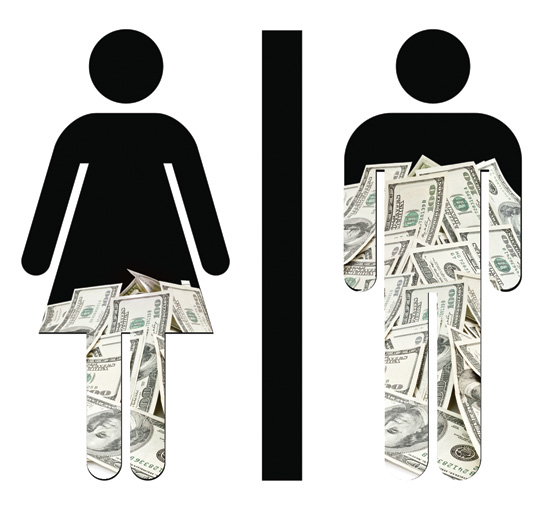## Accident occurrence

Suppose that accidents occurring on a highway each day can be assumed to be weakly dependent (essentially independent) and that the probability of an accident occurring is small. Assume that the random variable $$X$$ corresponding to the number of accidents occurring today has an expected value of three. What is the probability that exactly 2 accidents occur, given that it is known at least one accident occurs today?

$$X \sim Pois(\lambda = 3) \Rightarrow \mathbb{P}(X = x) = e^{-\lambda} \left(\dfrac{\lambda^x}{x!}\right) = e^{-3} \left(\dfrac{3^x}{x!}\right)$$

• Want to find $$\mathbb{P}[ (X = 2) \,|\, (X \ge 1)]$$

• Using the definition of conditional probability,

$\mathbb{P}[ (X = 2) \,|\, (X \ge 1)] = \dfrac{\mathbb{P} [ (X = 2) \text{ AND } (X \ge 1) ]}{\mathbb{P}(X \ge 1)} = \dfrac{\mathbb{P}(X = 2)}{\mathbb{P}(X \ge 1)}$

$$= \dfrac{\mathbb{P}(X = 2)}{1 - \mathbb{P}(X = 0)} = \dfrac{e^{-3}\left( \dfrac{3^2}{2!}\right)}{1 - \left[e^{-3}\left( \dfrac{3^0}{0!}\right)\right]} \approx \dfrac{0.224}{0.950} = 0.236$$

## Gender Discrimination• 48 male supervisors given the same personnel file.
• Files were identical except gender.
• Files were randomly assigned to the supervisors.

Question of interest: are females unfairly discriminated against in terms of promotions given by male supervisors?

## Data

Gender promote nopromote
Male 21 3
Female 14 10

$\mathbb{P}(\text{promote}\,|\,M) = 21/24 = 0.875 \\ \mathbb{P}(\text{promote}\,|\,F) = 14/24 = 0.583$

At a first glance, does there appear to be a relationship between promotion and gender?

## Question

We saw a difference of almost 30% (29.2% to be exact) between the proportion of male and female files that are promoted. Based on this information, which of the below is true?

1. If we were to repeat the experiment we will definitely see that more female files get promoted. This was a fluke.
2. Promotion is dependent on gender, males are more likely to be promoted, and hence there is gender discrimination against women in promotion decisions.
3. The difference in the proportions of promoted male and female files is due to chance, this is not evidence of gender discrimination against women in promotion decisions.
4. Women are less qualified than men, and this is why fewer females get promoted.

## Two competing claims

$$H_0$$, Null Hypothesis: "There is nothing going on."

• Promotion and gender are independent.
• No gender discrimination.
• Observed difference in proportions is simply due to chance.

$$H_a$$, Alternative Hypothesis: "There is something going on.”

• Promotion and gender are dependent.
• There is gender discrimination.
• Observed difference in proportions is not due to chance.
• $$\mathbb{P}(promote\,|\,M) > \mathbb{P}(promote\,|\,F)$$